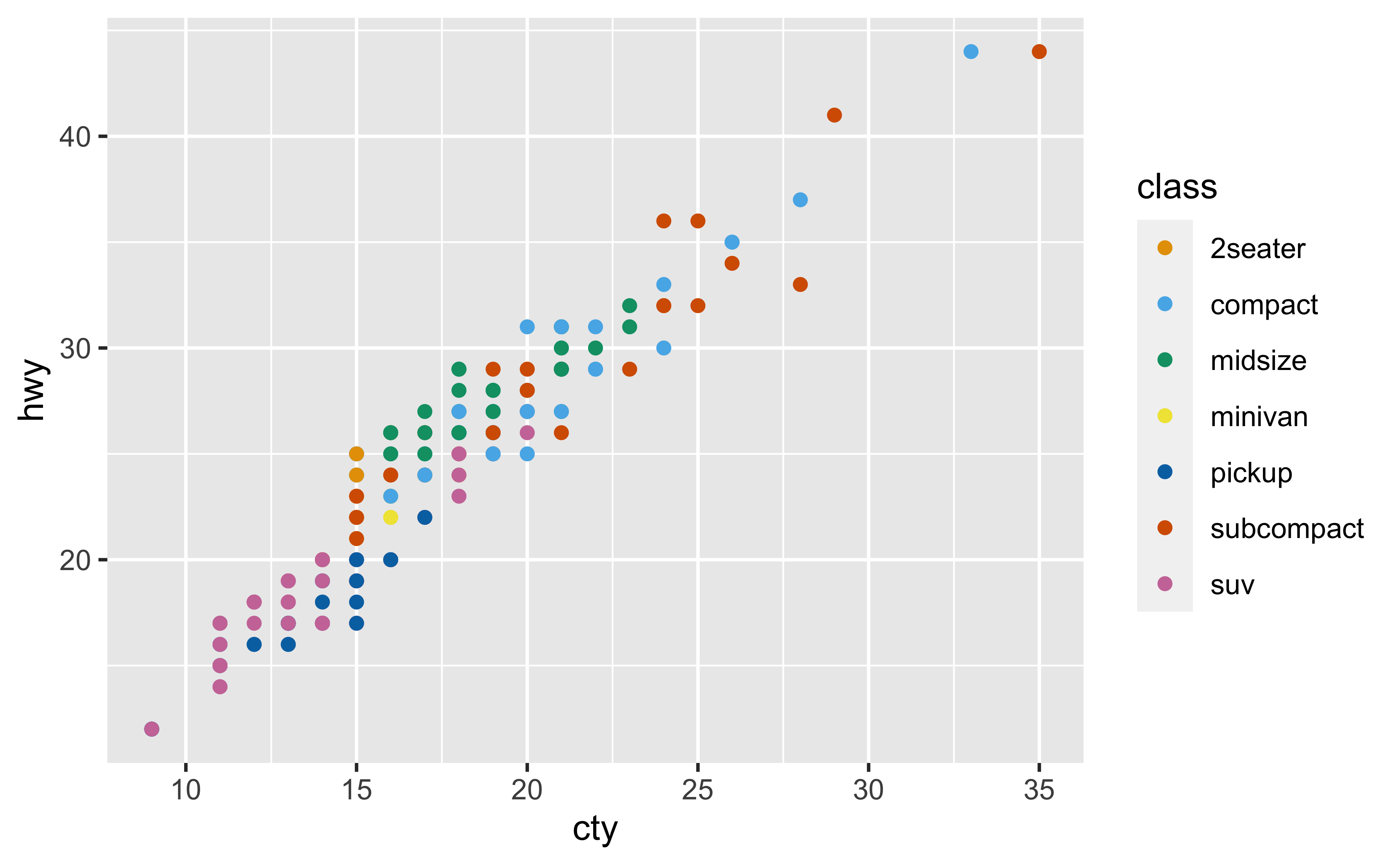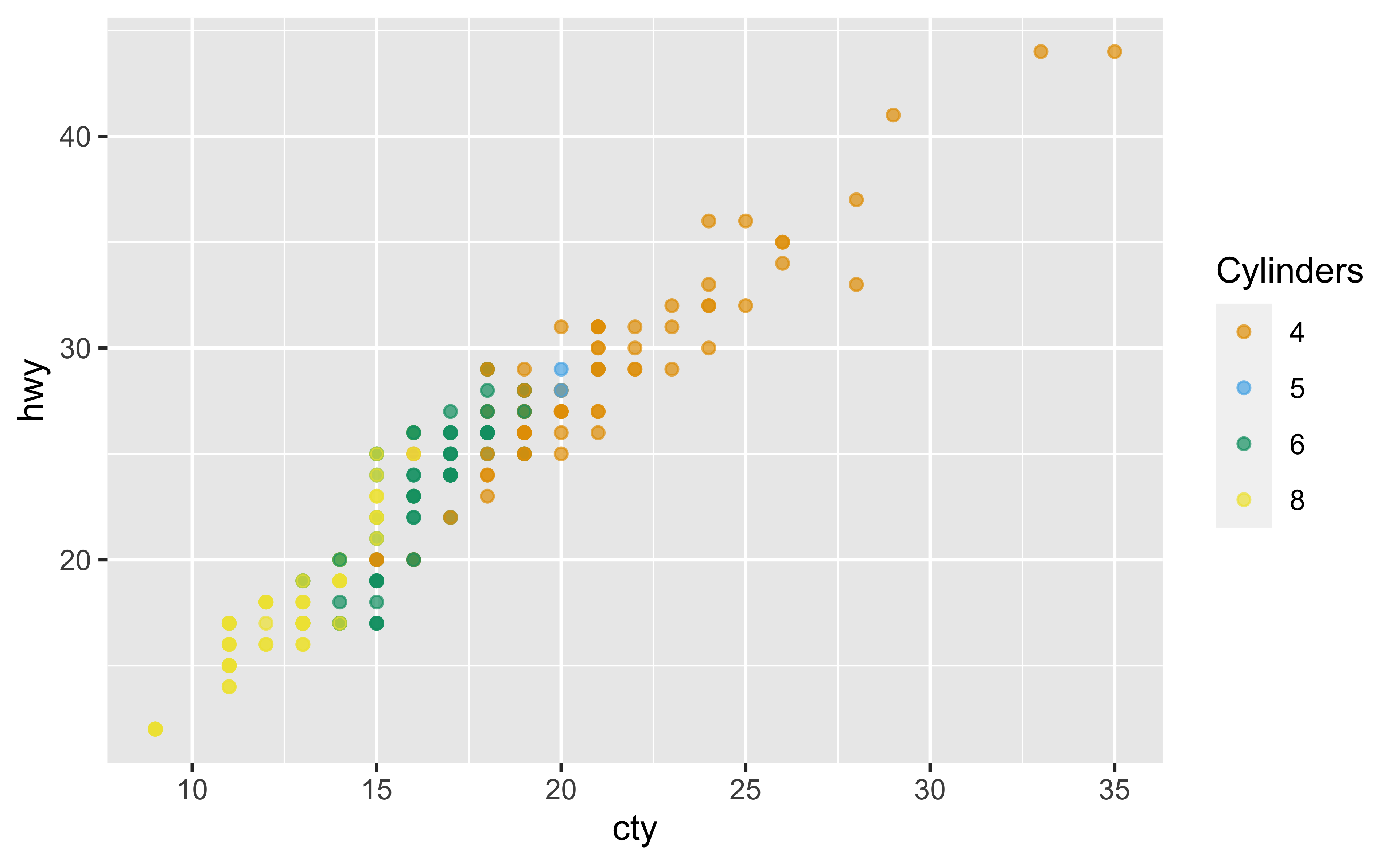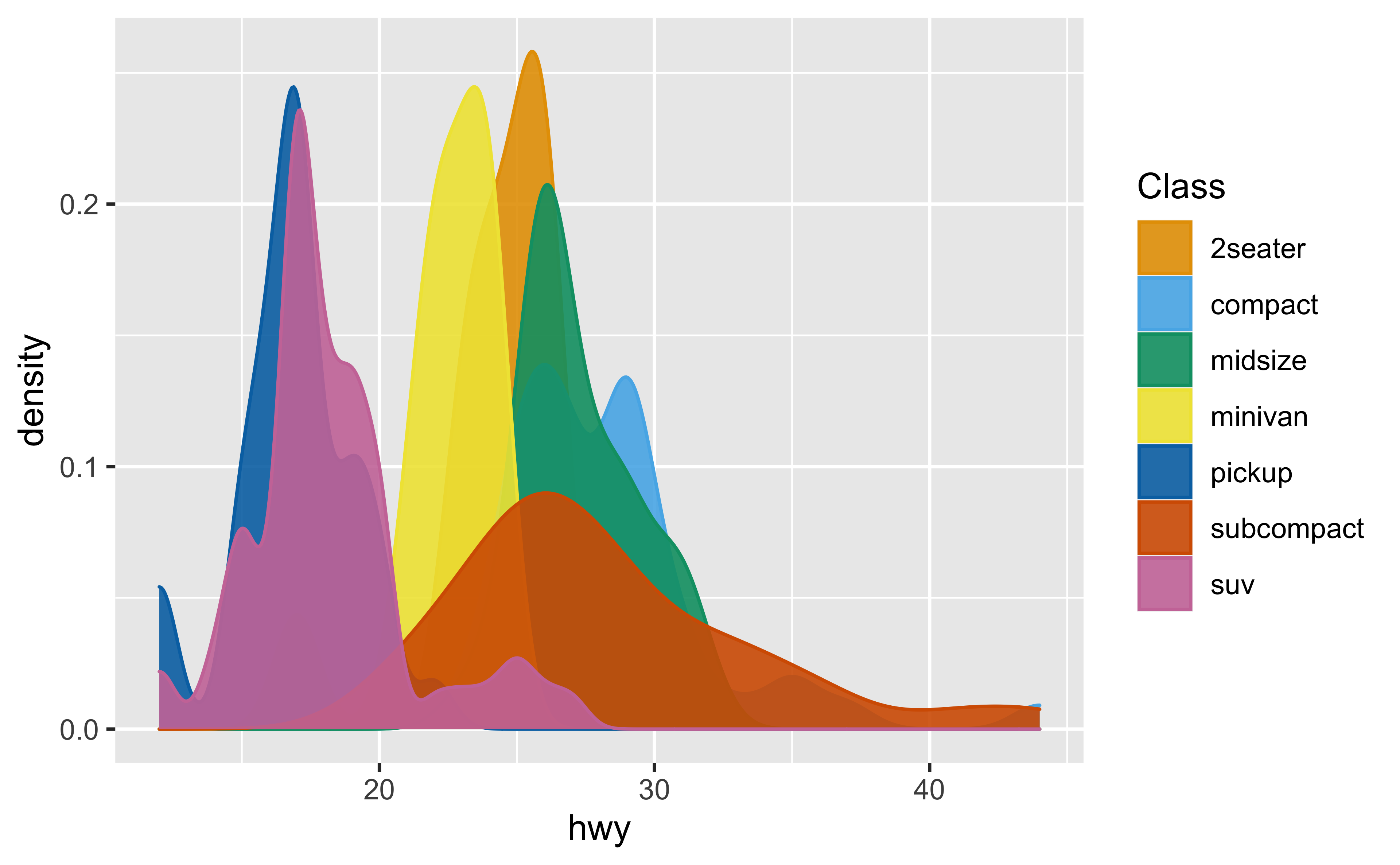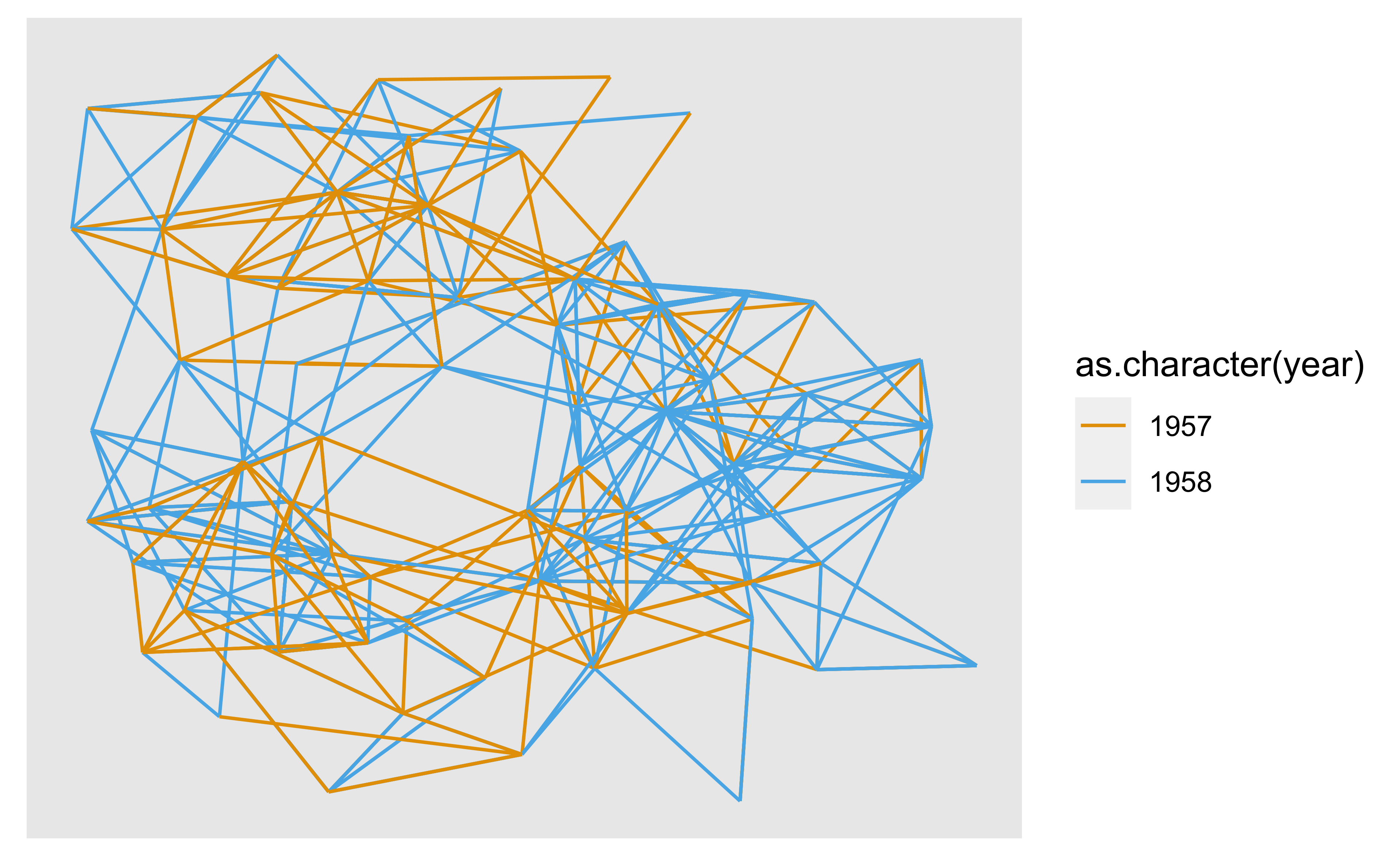ggokabeito provides ggplot2 and ggraph scales to easily use the discrete, colorblind-friendly ‘Okabe-Ito’ palette in your data visualizations. Currently, ggokabeito provides the following scales:

• scale_color_okabe_ito()/scale_colour_okabe_ito()
• scale_fill_okabe_ito()
• scale_edge_color_okabe_ito()/scale_edge_colour_okabe_ito()

## Installation

You can install ggokabeito from CRAN with:

install.packages("ggokabeito")

You can alternatively install the development version of ggokabeito from GitHub with:

# install.packages("devtools")
devtools::install_github("malcolmbarrett/ggokabeito")

## Examples

library(ggokabeito)
library(ggplot2)

ggplot(mpg, aes(cty, hwy, color = class)) +
geom_point() +
scale_color_okabe_ito()ggplot(mpg, aes(cty, hwy, color = factor(cyl))) +
geom_point(alpha = 0.7) +
scale_color_okabe_ito(name = "Cylinders", alpha = .9)ggplot(mpg, aes(hwy, color = class, fill = class)) +
geom_density() +
scale_fill_okabe_ito(name = "Class", alpha = .9) +
scale_color_okabe_ito(name = "Class")ggokabeito also works with ggraph

# example from https://www.data-imaginist.com/2017/ggraph-introduction-edges/
library(ggraph, warn.conflicts = FALSE)
library(igraph, warn.conflicts = FALSE)

graph <- graph_from_data_frame(highschool)
pop1957 <- degree(
delete_edges(graph, which(E(graph)$year == 1957)), mode = "in" ) pop1958 <- degree( delete_edges(graph, which(E(graph)$year == 1958)),
mode = "in"
)
V(graph)$pop_devel <- ifelse( pop1957 < pop1958, "increased", ifelse(pop1957 > pop1958, "decreased", "unchanged" ) ) V(graph)$popularity <- pmax(pop1957, pop1958)
E(graph)$year <- as.character(E(graph)$year)

ggraph(graph, layout = "kk") +
scale_edge_color_okabe_ito()